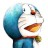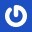# 杰拉斯的博客

## [ACM_Tyvj_1011]传纸条（双线程动态规划解法）杰拉斯 | 时间：2013-05-09, Thu | 44,917 views

### 传纸条

```3 3
0 3 9
2 8 5
5 7 0
```

```34
```

#### 来源

Tyvj1011

1. 第一个点由上走来，第二个点也由上走来，此时的好感度和为f[i - 1][j][k - 1][l] + a[i][j] + a[k][l]
2. 第一个点由上走来，第二个点则由左走来，此时的好感度和为f[i - 1][j][k][l - 1] + a[i][j] + a[k][l]，但此时应考虑第一个点的上方的点是否会与第二个点的左方的点重合，如果重合则不可取
3. 第一个点由左走来，第二个点则由上走来，此时的好感度和为f[i][j - 1][k - 1][l] + a[i][j] + a[k][l]，但此时应考虑第一个点的左方的点是否会与第二个点的上方的点重合，如果重合则不可取
4. 第一个点由左走来，第二个点也由左走来，此时的好感度和为f[i][j - 1][k][l - 1] + a[i][j] + a[k][l]

```#include<stdio.h>

int a = {0};
// 好感度数组
int f = {0};
// 动态规划数组，f[i][j][k][l] 表示从 (0, 0) 位置由两条不交叉的线路走到 (i, j)，(k, l) 位置时的最大好感度和

int max(int a, int b){
return a > b ? a : b;
}

int main(){
int m, n;
scanf("%d%d", &m, &n);
for(int i = 1; i <= m; ++i){
for(int j = 1; j <= n; ++j){
scanf("%d", &a[i][j]);
}
}
// 开始循环解题
for(int i = 1; i <= m; ++i){
for(int j = 1; j <= n; ++j){
for(int k = 1; k <= m; ++k){
for(int l = 1; l <= n; ++l){
// 还没到终点前不能走到同一个点，因此(i, j)不能等于(k, l)
// 加上小于判断是因为当(i, j)跟(k, l)互换时，最大好感度值必定一样，不必重复计算
if((i < m || j < n) && i <= k && j <= l){
continue;
}
int num = 0;
// 两个点都由上走来的好感度
num = max(num, f[i - 1][j][k - 1][l]);
// 第一个点由上走来，第二个点从左走来，并且两个来源点不重合时的好感度
if(i - 1 != k && j != l - 1){
num = max(num, f[i - 1][j][k][l - 1]);
}
// 第一个点由左走来，第二个点从上走来，并且两个来源点不重合时的好感度
if(i != k - 1 && j - 1 != l){
num = max(num, f[i][j - 1][k - 1][l]);
}
// 两个点都由左走来的好感度
num = max(num, f[i][j - 1][k][l - 1]);
// 加上当前两点的好感度，即为走到这两点时的最大好感度和
f[i][j][k][l] = num + a[i][j] + a[k][l];
}
}
}
}
// 输出由两条不交叉的线路走到右下角时的最大好感度和
printf("%d\n", f[m][n][m][n]);
return 0;
}
```

#### 2 条评论 »

1.noname

i+j = k+l 恒成立

2.lunettes oakley

[ACM_Tyvj_1011]传纸条（双线程动态规划解法） - 蓝飞技术部落格 —— 关注前沿，追求卓越。 oakley www.87wg.com
lunettes oakley http://www.87wg.com/